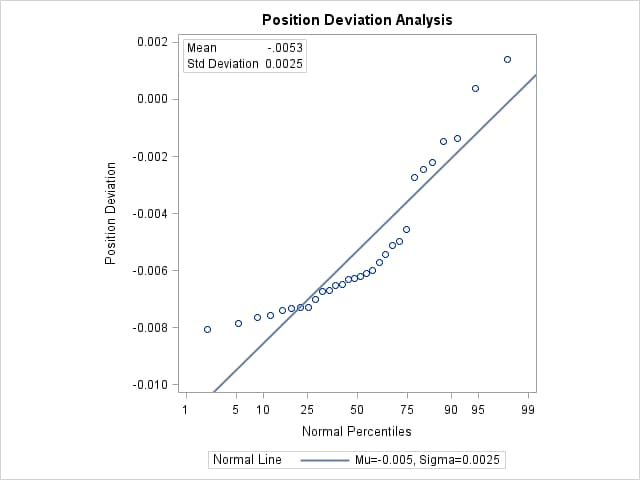# The UNIVARIATE Procedure

### Modeling a Data Distribution

In addition to summarizing a data distribution as in the preceding example, you can use PROC UNIVARIATE to statistically model a distribution based on a random sample of data. The following statements create a data set named `Aircraft` that contains the measurements of a position deviation for a sample of 30 aircraft components.

```data Aircraft;
input Deviation @@;
label Deviation = 'Position Deviation';
datalines;
-.00653 0.00141 -.00702 -.00734 -.00649 -.00601
-.00631 -.00148 -.00731 -.00764 -.00275 -.00497
-.00741 -.00673 -.00573 -.00629 -.00671 -.00246
-.00222 -.00807 -.00621 -.00785 -.00544 -.00511
-.00138 -.00609 0.00038 -.00758 -.00731 -.00455
;

```

An initial question in the analysis is whether the measurement distribution is normal. The following statements request a table of moments, the tests for normality, and a normal probability plot, which are shown in Figure 4.5 and Figure 4.6:

```title 'Position Deviation Analysis';
ods graphics on;
ods select Moments TestsForNormality ProbPlot;
proc univariate data=Aircraft normaltest;
var Deviation;
probplot Deviation / normal(mu=est sigma=est)
square
odstitle = title;
label Deviation = 'Position Deviation';
inset  mean std / format=6.4;
run;
```

PROC UNIVARIATE uses the label associated with the variable `Deviation` as the vertical axis label in the probability plot. The INSET statement displays the sample mean and standard deviation on the probability plot.

Figure 4.5: Moments and Tests for Normality

 Position Deviation Analysis

The UNIVARIATE Procedure
Variable: Deviation (Position Deviation)

Moments
N 30 Sum Weights 30
Mean -0.0053067 Sum Observations -0.1592
Std Deviation 0.00254362 Variance 6.47002E-6
Skewness 1.2562507 Kurtosis 0.69790426
Uncorrected SS 0.00103245 Corrected SS 0.00018763
Coeff Variation -47.932613 Std Error Mean 0.0004644

Tests for Normality
Test Statistic p Value
Shapiro-Wilk W 0.845364 Pr < W 0.0005
Kolmogorov-Smirnov D 0.208921 Pr > D <0.0100
Cramer-von Mises W-Sq 0.329274 Pr > W-Sq <0.0050
Anderson-Darling A-Sq 1.784881 Pr > A-Sq <0.0050

All four goodness-of-fit tests in Figure 4.5 reject the hypothesis that the measurements are normally distributed.

Figure 4.6 shows a normal probability plot for the measurements. A linear pattern of points following the diagonal reference line would indicate that the measurements are normally distributed. Instead, the curved point pattern suggests that a skewed distribution, such as the lognormal, is more appropriate than the normal distribution.

A lognormal distribution for `Deviation` is fitted in Example 4.26.

A sample program for this example, univar2.sas, is available in the SAS Sample Library for Base SAS software.

Figure 4.6: Normal Probability Plot